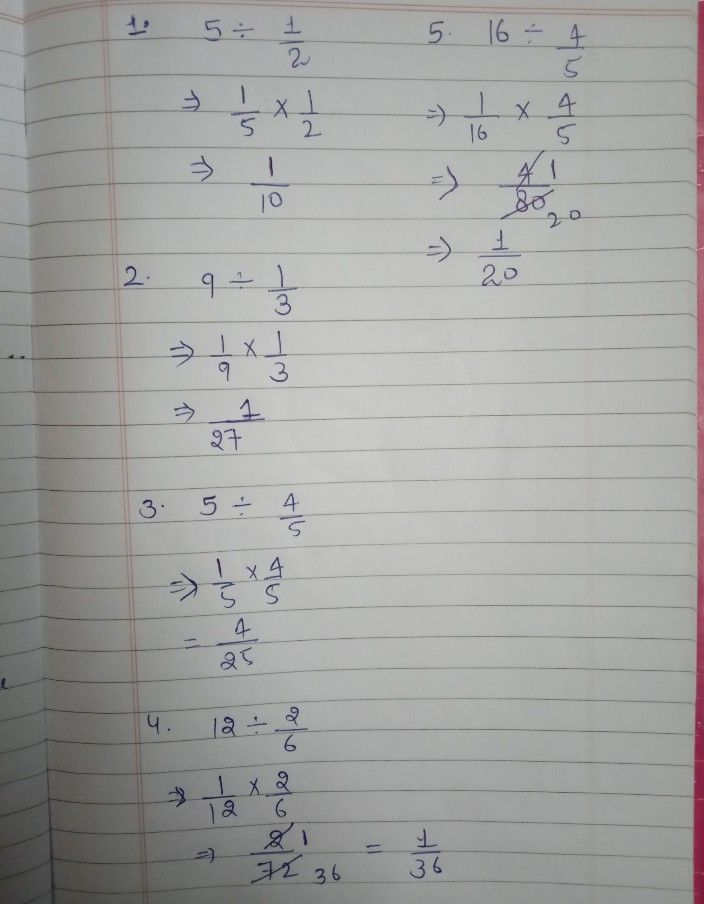Symbol
ProblemLearning Task $2$ Find the quotient. Reduce your answer to lowest te Write your answer in your notebook. $1$ $5$ 4] $1$ 4. $2$ $12$ $4$ $2$ $6$ $2$ 4. $0$ $1$ $5$ $16$ $5$ $3$ $3$ $8$ 4. $5$
1st-6th grade
Other
Search count: 105
Question content
find the quotient
SolutionQanda teacher - sufiamalik